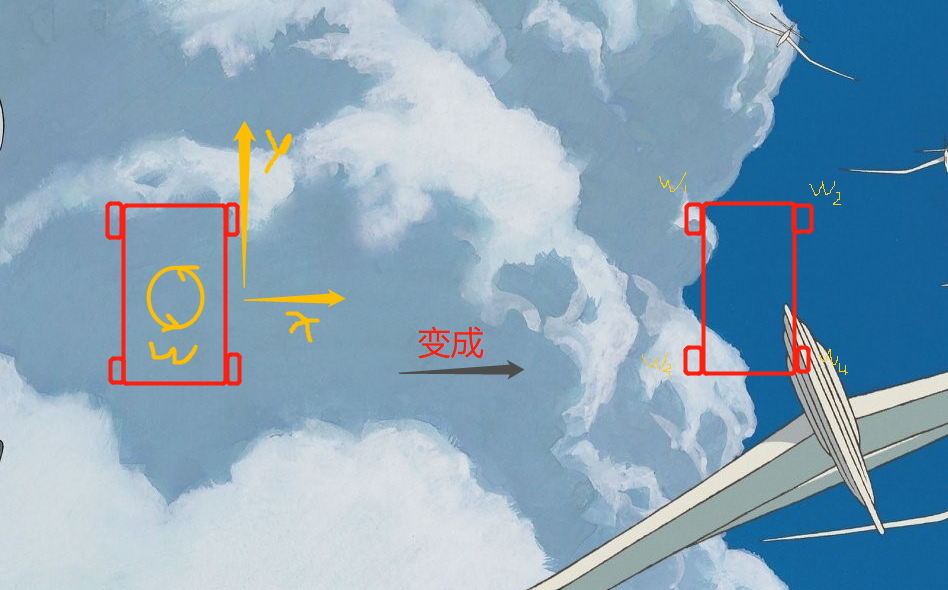##用户名 Email 自动登录 找回密码 密码 立即注册

# 关于51单片机驱动麦克那姆轮的调速问题a214562573 发表于 2019-5-26 17:36 | 显示全部楼层 |阅读模式
3黑币
 如题 最近在做一个基于51单片机的麦轮遥控小车。由于是第一次接触麦轮，一开始我想的是不需要调速，能前进后退平移就行了。但是程序写好后朋友告诉我说如果不进行调速，小车并不会走直线，反而会倾斜前进。所以我想问下如何对麦轮进行调速控制，以及为什么需要调速。谢谢！ 51单片机的程序在下面 如果可以的话，能帮我讲解下需要如何调整 #define uint unsigned int #define uchar unsigned char sbit out1=P2^0; sbit out2=P2^1; sbit out3=P2^2; sbit out4=P2^3; sbit out5=p2^4; sbit out6=p2^5; sbit out7=p2^6; sbit out8=p2^7; void qianjin()//前进 { out1=0;out2=1; out3=0;out4=1; out5=0;out6=1; out7=0;out8=1； } void houtui()//后退 { out1=1;out2=0; out3=1;out4=0; out5=1;out6=0; out7=1;out8=0; } void zuozhuan()//左转 { out1=0;out2=0; out3=0;out4=1; out5=0;out6=0; out7=0;out8=1; } void youzhuan()//右转 { out1=0;out2=1; out3=0;out4=0; out5=0;out6=1; out7=0;out8=0; } void tingzhi()//停止 { out1=0;out2=0; out3=0;out4=0; out5=0;out6=0; out7=0;out8=0; } void init()         { IE=0x90; SCON=0x50; TMOD=0x20; TH1=0xfd; TL1=0xfd; TCON=0x40; TR1=1; ES = 1; EA=1; } void main() { init(); while(1) { } } void time() interrupt 4 {                                                                                                                                                                                               if(RI) { RI=0; switch(SBUF) { case 'a':qianjin();break; case 'b':houtui();break; case 'c':zuozhuan();break; case 'd':youzhuan();break; case 'e':tingzhi();break; }                                                                                                          } }

### 最佳答案liushangming 发表于 2019-5-26 17:36 | 显示全部楼层
 你朋友的调速是指通过检测实际速度进行反馈调节，你可以去了解一PID速度控制楼主| a214562573 发表于 2019-5-27 09:42 | 显示全部楼层
 liushangming 发表于 2019-5-27 08:50 你朋友的调速是指通过检测实际速度进行反馈调节，你可以去了解一PID速度控制 就是用电机的编码器进行调速吗？不需要在程序中修改什么太空将军 发表于 2019-6-3 11:34 | 显示全部楼层
 我发表过的》》麦克纳姆轮全向轮战车程序精髓分享《《    自己搜索看看 因为：由于麦克轮是将(X,Y,W)转换成(W1,W2,W3,W4) 翻译：X为横着走的速度，Y为前进走速度，W为原地转速度。W1,W2,W3,W4为各个轮子速度捷径：如果你只是玩耍，不是做项目，那么只需要简单的控制4个电机，不断地试验电机组合，看看不同轮子转向得到什么结果，就能总结出规律

 本版积分规则 回帖并转播 回帖后跳转到最后一页